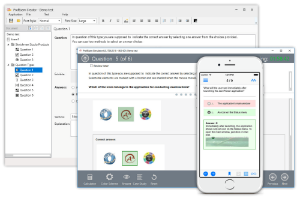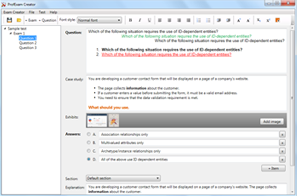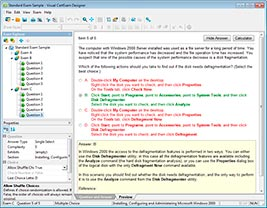## File Info

 Exam Introduction to Programming Using Java Number 98-388 File Name Introduction to Programming Using Java.CertDumps.98-388.2019-09-01.1e.21q.vcex Size 1.55 Mb Posted September 01, 2019 Downloads 161

### How to open VCEX & EXAM Files?

Files with VCEX & EXAM extensions can be opened by ProfExam Simulator.

Coupon: EXAMFILESCOM
With discount: 20%

## Demo Questions

Question 1
You have the following code segment. Line numbers are included for reference only.What is the output of line 07?

• A: -44
• B: -40.0
• C: 40.0
• D: 44.0

Question 2
You need to analyze the following code segment. Line numbers are included for reference only.What is the output of line 12 when you run printInt()?

• A: 0
• B: 1
• C: 2
• D: 3

Question 3
You are creating a Java console application.
You need to read a birthdate entered by the user.
How should you complete the code? To answer, select the appropriate code segments in the answer area.
NOTE: Each correct selection is worth one point.

Question 4
You need to evaluate the following Java program. Line numbers are included for reference only.Which three values will be displayed in sequence? To answer, move the appropriate values from the list of values to the answer area and arrange them in the
correct order.
NOTE: Each correct selection is worth one point.

Question 5
You need to evaluate the following code. Line numbers are included for reference only.Use the drop-down menus to select the answer choice that answers each question based on the information presented in the code.
NOTE: Each correct selection is worth one point.

Question 6
You have the following code:You need to create an int array named numbers initialized with num1, num2, and num3.
How should you complete the code? To answer, drag the appropriate code segment to the correct position. Each code segment may be used once, more than
once, or not at all. You may need to drag the split bar between panes or scroll to view content.
NOTE: Each correct selection is worth one point.Question 7
You are writing a Java program.
The program must meet the following requirements:
Truncate firstName to its first five characters
Set output to a string that contains the firstName and the number of characters in firstName
How should you complete the code? To answer, select the appropriate code segments in the answer area.
NOTE: Each correct selection is worth one point.

Question 8
The question requires that you evaluate the underlined text to determine if it is correct.
You should use an int data type to store the numeric value 3,000,000,000 (3 billion) so that the least amount of memory is used.
Review the underlined text. If it makes the statement correct, select “No change is needed.” If the statement is incorrect, select the answer choice that makes the statement correct.

• A: No change is needed.
• B: a short
• C: a byte
• D: a long

Question 9
You need to evaluate the following code segment:What happens when the code segment is run?

• A: iNum has a value of 0.
• B: An exception is thrown.
• C: iNum has a value of 2.
• D: iNum has a value of 3.

Question 10
You are writing a Java method.
The program must meet the following requirements:
Accept a String parameter firstName
Display a welcome message that contains firstName
Ensure that the first letter of the name is capitalized, and the remaining letters are in lowercase
How should you complete the code? To answer, drag the appropriate code segment to the correct position. Each code segment may be used once, more than
once, or not at all. You may need to drag the split bar between panes or scroll to view content.
NOTE: Each correct selection is worth one point.

CONNECT US##### PROFEXAM WITH A 20% DISCOUNT

You can buy ProfExam with a 20% discount..

HOW TO OPEN VCEX AND EXAM FILES

Use ProfExam Simulator to open VCEX and EXAM filesHOW TO OPEN VCE FILES

Use VCE Exam Simulator to open VCE files